In Online Education NCERT Solutions for Class 8 Maths Chapter 11 Mensuration Ex 11.2 are part of NCERT Solutions for Class 8 Maths. Here we have given NCERT Solutions for Class 8 Maths Chapter 11 Mensuration Ex 11.2.

 Board CBSE Textbook NCERT Class Class 8 Subject Maths Chapter Chapter 11 Chapter Name Mensuration Exercise Ex 11.2 Number of Questions Solved 11 Category NCERT Solutions

## NCERT Solutions for Class 8 Maths Chapter 11 Mensuration Ex 11.2

Question 1.
The shape of the top surface of a table is a trapezium. Find its area, if its parallel sides are 1 m and 1.2 man the d perpendicular distance between them is 0.8 m.
Solution.
Area of the top surface of the table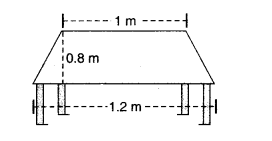= $$\frac { 1 }{ 2 } h(a+b)$$
= $$\frac { 1 }{ 2 } \times 0.8\times (1.2+1)$$
= $$0.88{ m }^{ 2 }$$

Question 2.
The area of a trapezium is 34 cm2 and the length of one of the parallel sides is 10 cm and its height is 4 cm. Find the length of the another parallel side.
Solution.
Area of trapezium
= $$\frac { 1 }{ 2 } h(a+b)$$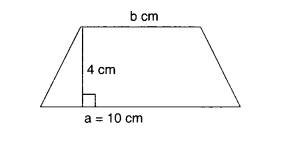⇒ $$34=\frac { 1 }{ 2 } \times 4(10+b)$$
⇒ $$34=2\times (10+b)$$
⇒ $$10+b=\frac { 34 }{ 2 }$$
⇒ 10 + b=17
⇒ b = 17 – 10
⇒ b = 7 cm
Hence, the length of another parallel side is 7 cm.

Question 3.
Length of the fence of a trapezium shaped field ABCD is 120 m. If BC = 48 m, CD = 17 m and AD = 40 m, find the area of this field. Side AB is perpendicular to the parallel sides AD and BCSolution.
Fence of the trapezium shaped field ABCD = 120 m
⇒ AB + BC + CD + DA = 120
⇒ AB + 48 + 17 + 40 = 120
⇒ AB + 105 = 120
⇒ AB = 120 – 105
⇒ AB = 15 m
∴ Area of the field
= $$\frac { (BC+AD)\times AB }{ 2 }$$
= $$\frac { (48+40)\times 16 }{ 2 }$$ = 660 $${ m }^{ 2 }$$

Question 4.
The diagonal of a quadrilateral shaped field is 24 m and the perpendiculars dropped on it from the remaining opposite vertices are 8 m and 13 m. Find the area of the field.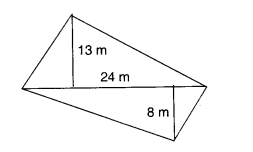Solution.
Area of the field
= $$\frac { 1 }{ 2 } d({ h }_{ 1 }+{ h }_{ 2 })$$
= $$\frac { 24\times (8+13) }{ 2 }$$ = $$\frac { 24\times 21 }{ 2 }$$
= 12 x 21 = 252$${ m }^{ 2 }$$

Question 5.
The diagonals of a rhombus are 7.5 cm and 12 cm. Find its area.
Solution.
Area of the rhombus= $$\frac { 1 }{ 2 } \times { d }_{ 1 }\times { d }_{ 2 }$$
= $$\frac { 1 }{ 2 } \times 7.5\times 12$$
= 45 $${ m }^{ 2 }$$

Question 6.
Find the area of a rhombus whose side is 5 cm and whose altitude is 4.8 cm. If one of its diagonals is 8 cm long, find the length of the other diagonal.
Solution.
Area of the rhombus
= base (b) x altitude (h) = 5
= 5 x 4.8 = 24 $${ cm }^{ 2 }$$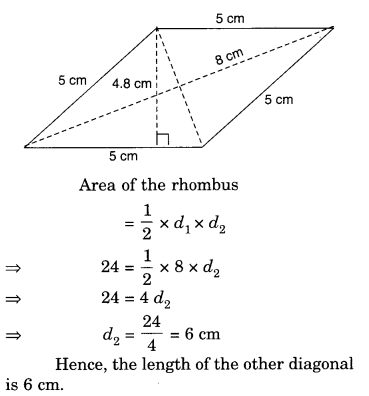Question 7.
The floor of a building consists of 3,000 tiles which are rhombus shaped and each of its diagonals are 45 cm and 30 cm in length. Find the total cost of polishing the floor, if the cost per $${ m }^{ 2 }$$ is ₹ 4.
Solution.
Area of a tileQuestion 8.
Mohan wants to buy a trapezium shaped field. Its side along the river is parallel to and twice the side along the road. If the area of this field is 10,500 $${ m }^{ 2 }$$ and the perpendicular distance between the two parallel sides is 100 m, find the length of the side along the river.
Solution.
Let the length of the side along the road be x m. Then, the length of the side along the river is 2x m.
Area of the field = 10,500 square metres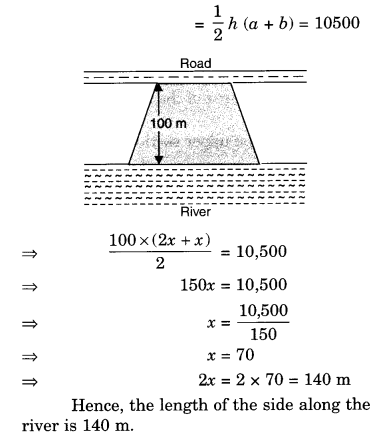Question 9.
Top surface of a raised platform is in the shape of a regular octagon as shown in the figure. Find the area of the octagonal surface.
Solution.
Area of the octagonal surface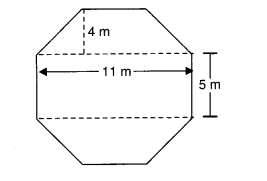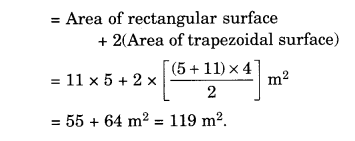Question 10.
There is a pentagonal shaped park as shown in the figure. For finding its area Jyoti and Kavita divided it in two different ways.Find the area of this park using both ways. Can you suggest some other way of finding its area ?
Solution.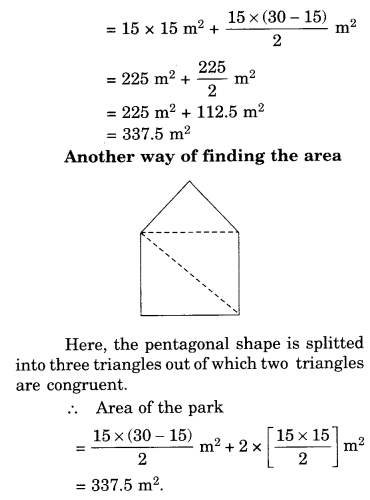Question 11.
Diagram of the adjacent picture frame has outer dimensions = 24 cm x 28 cm and inner dimensions 16 cm x 20 cm. Find the area of each section of the frame, if the width of each section the same.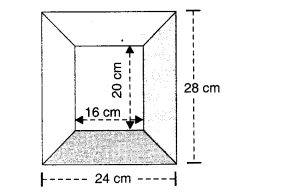Solution.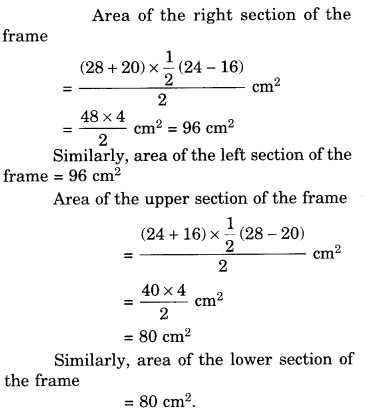We hope the NCERT Solutions for Class 8 Maths Chapter 11 Mensuration Ex 11.2 help you. If you have any query regarding NCERT Solutions for Class 8 Maths Chapter 11 Mensuration Ex 11.2, drop a comment below and we will get back to you at the earliest.